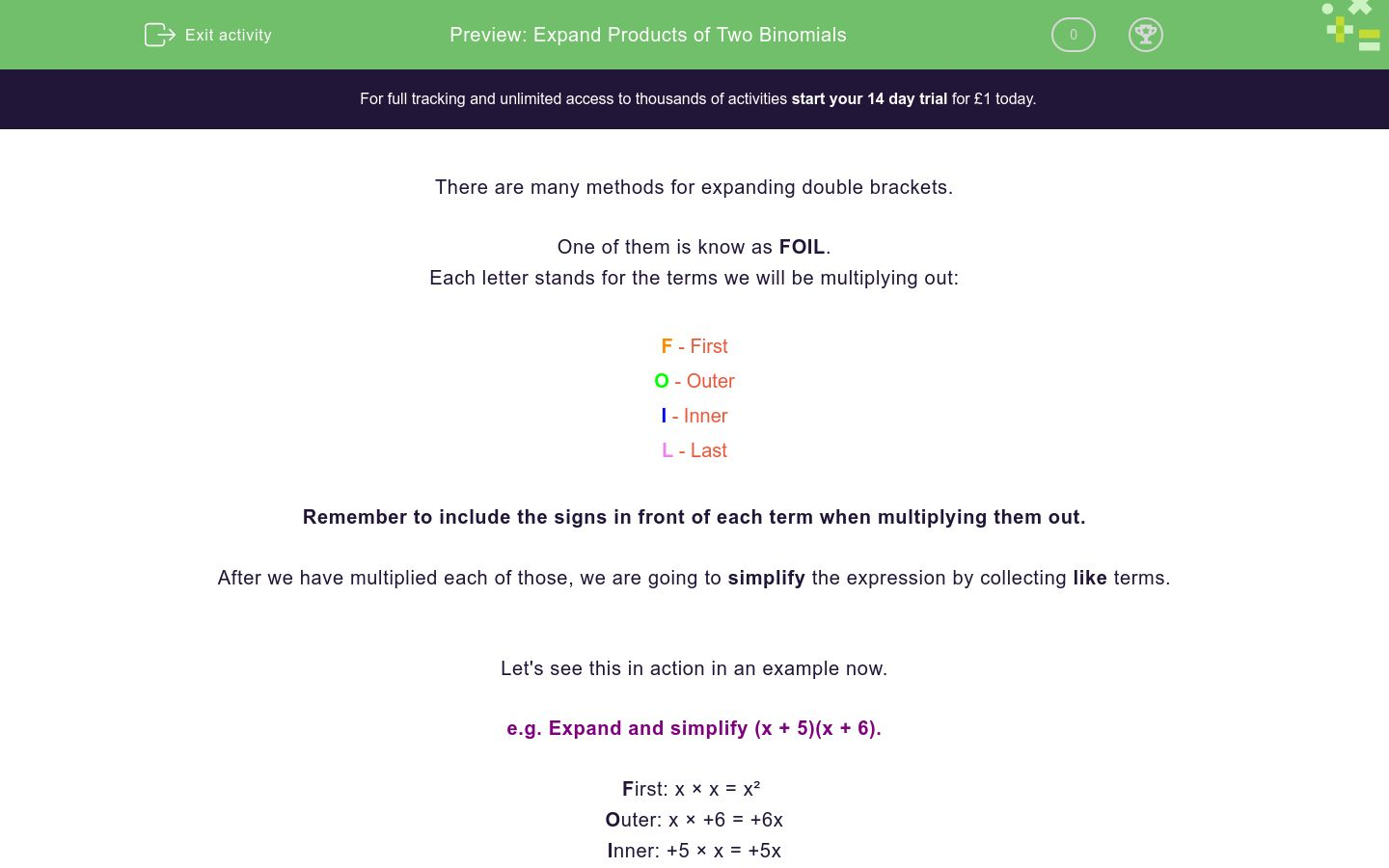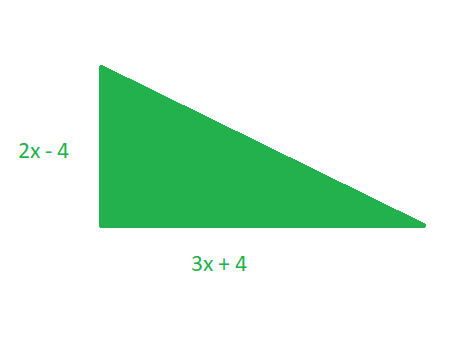# Expand Products of Two Binomials

In this worksheet, students will use the FOIL (First - Outer - Inner - Last) method to expand and simplify double brackets.Key stage:  KS 4

GCSE Subjects:   Maths

GCSE Boards:   Pearson Edexcel, OCR, Eduqas, AQA

Curriculum topic:   Algebra

Curriculum subtopic:   Notation, Vocabulary and Manipulation, Algebraic Expressions

Difficulty level:### QUESTION 1 of 10

There are many methods for expanding double brackets.

One of them is know as FOIL.

Each letter stands for the terms we will be multiplying out:

### L - Last

Remember to include the signs in front of each term when multiplying them out.

After we have multiplied each of those, we are going to simplify the expression by collecting like terms.

Let's see this in action in an example now.

e.g. Expand and simplify (x + 5)(x + 6).

First: x × x = x²

Outer: x × +6 = +6x

Inner: +5 × x = +5x

Last: +5 × +6 = +30

Now let's simplify by collecting like terms (highlighted):

x² + 6x + 5x + 30 = x² + 11x + 30

That wasn't so difficult, was it?

In this activity, you will expand and simplify two sets of brackets using the FOIL method shown above.

You may want to have a pen and paper handy so that you can record your working.

Expand and simplify:

(x + 2)(x + 3)

x² + 6

x² + 11x

x² + 5x + 6

12x²

Eric tried using the foil method to expand (2y + 4)(3y - 5).

Here are his workings:

F: 2y × 3y = 5y

O: 2y × -5 = -10y

I: 4 × 3y = 12y

L: 4 × -5 = -1

How many mistakes did Eric make?

0 mistakes

1 mistake

2 mistakes

3 mistakes

Expand and simplify:

(x + 8)(x + 5)

14x² + 40

x² + 40x + 13

x² + 13x +13

x² + 13x + 40

Match each set of double brackets with their expanded form.

## Column B

(x - 2)(x + 7)
x² - 9x + 14
(x + 2)(x + 7)
x² + 9x + 14
(x + 2)(x - 7)
x² - 5x - 14
(x - 2)(x - 7)
x² + 5x - 14

Expand and simplify:

(x - 2)²

x² - 4

x² + 4

x² - 4x + 4

x² + 4x + 4

Which expressions from the options below can be used to calculate the area of this rectangle?(a + 7)(2a + 3)

3a + 10

2a² + 17a + 21

2a² + 21

Your friend has been working on expanding:

(x + 7)(x + 7)

They have used the FOIL method and then written their answer below.

One of their terms is incorrect.

Which is it?

x² + 14x + 14

Which expressions from the options below can be used to calculate the area of this triangle?(3x + 4)(2x - 4)

0.5(3x + 4)(2x - 4)

6x² - 4x - 16

3x² - 2x - 8

Match each of the brackets below to their expanded versions.

## Column B

(x - 6)²
x² + 7x + 6
(x - 6)(x + 6)
x² + 12x + 36
(x + 6)²
x² - 12x + 36
(x + 6)(x + 1)
x² - 36

Your friend has been working on expanding:

(3x + 6)(2x - 5)

They have used the FOIL method and then written their answer below.

One of their terms is incorrect.

Which is it?

5x² - 3x - 30
• Question 1

Expand and simplify:

(x + 2)(x + 3)

x² + 5x + 6
EDDIE SAYS
Did you use the FOIL method? First: x × x = x² Outer: x × 3 = 3x Inner: 2 × x = 2x Last: 2 × 3 = 6 Now put these all together and simplify: x² + 3x + 2x + 6 = x² + 5x + 6
• Question 2

Eric tried using the foil method to expand (2y + 4)(3y - 5).

Here are his workings:

F: 2y × 3y = 5y

O: 2y × -5 = -10y

I: 4 × 3y = 12y

L: 4 × -5 = -1

How many mistakes did Eric make?

2 mistakes
EDDIE SAYS
Eric made two mistakes. The first one was when he was multiplying out 'First' terms of the brackets. The correct working should read: F: 2y × 3y = 6y² He added them instead of multiplying them! The second mistake is in the last line of his working. He did not multiply the terms, but added them. The correct working should be: L: 4 × -5 = -20 If Eric had not made these errors, he would have arrived at the correct answer, which is: 6y2 + 2y - 20
• Question 3

Expand and simplify:

(x + 8)(x + 5)

x² + 13x + 40
EDDIE SAYS
The last option is correct, as it shows that a correct method for expanding and simplifying was used. Let's have a look and follow this method through: F: x × x = x² O: x × +5 = +5x I: +8 × x = +8x L: +8 × +5 = +40 Now we need to simplify: x² + 5x + 8x + 40 This gives us an answer of: x² + 13x + 40
• Question 4

Match each set of double brackets with their expanded form.

## Column B

(x - 2)(x + 7)
x² + 5x - 14
(x + 2)(x + 7)
x² + 9x + 14
(x + 2)(x - 7)
x² - 5x - 14
(x - 2)(x - 7)
x² - 9x + 14
EDDIE SAYS
Did you use FOIL to work out each pair individually? You needed to be really careful with the positive and negative symbols here, as many of the terms were very similar. Let's compare (x + 2)(x - 7) and (x - 2)(x - 7), where there is just one sign different. F: x × x = x2 O: x × -7 = -7x I: +2 × x OR -2 × x = 2x or -2x L: 2 × -7 OR -2 × -7 = -14 or 14 So our one sign difference, creates two very different answers when we put together and simplify: x2 - 7x + 2x - 14 = x2 - 5x - 14 OR x2 - 7x - 2x + 14 = x2 - 9x + 14
• Question 5

Expand and simplify:

(x - 2)²

x² - 4x + 4
EDDIE SAYS
Did you spot that this is really double brackets? Squaring means multiplying by itself, so (x - 2)² is the same as: (x - 2)(x - 2) Using FOIL to expand these brackets we get: x² - 2x - 2x + 4 = x² - 4x + 4
• Question 6

Which expressions from the options below can be used to calculate the area of this rectangle?(a + 7)(2a + 3)
2a² + 17a + 21
EDDIE SAYS
How do you find the area of a rectangle? That's right, multiply length by width. This rectangle's length is (a + 7) and its width is (2a + 3) so one of the correct options is simply: (a + 7)(2a + 3) However, we can also expand the brackets using the FOIL method. This will give us 2a² + 17a + 21, so this is also a correct answer. Did you spot both options there?
• Question 7

Your friend has been working on expanding:

(x + 7)(x + 7)

They have used the FOIL method and then written their answer below.

One of their terms is incorrect.

Which is it?

+ 14x + 14
EDDIE SAYS
14 is incorrect, it should be 49! If we follow the FOIL method, we get: F: x × x = x2 O: x × 7 = 7x I: 7 × x = 7x L: 7 × 7 = 49 not 14 As a shortcut, you could have quickly checked the numbers of each element against the question. As we are working with '7', all terms need to be multiples of 7 but the number on its own (the 'L' in FOIL) was clearly not the product of 7 × 7. Did you spot that shortcut?
• Question 8

Which expressions from the options below can be used to calculate the area of this triangle?0.5(3x + 4)(2x - 4)
3x² - 2x - 8
EDDIE SAYS
Another tricky one! Did you remember that to find the area of a triangle you need to multiply base and height and then divide by 2? As our base is (3x + 4) and our height is (2x - 4), one way to find the area would be to multiply these together and by a half: 0.5(3x + 4)(2x - 4) But we can also multiply out the brackets using the FOIL method to reach another alternative. Don't let the 0.5 before the brackets confuse you - just work through as normal and deal with that at the end. If we multiply out (3x + 4)(2x - 4) using FOIL, we reach: 6x2 - 12x + 8x - 16 = 6x2 - 4x - 16 Now let's multiply each of the terms by 0.5, to reach: 3x2 - 2x - 8 Great work!
• Question 9

Match each of the brackets below to their expanded versions.

## Column B

(x - 6)²
x² - 12x + 36
(x - 6)(x + 6)
x² - 36
(x + 6)²
x² + 12x + 36
(x + 6)(x + 1)
x² + 7x + 6
EDDIE SAYS
Hopefully you remembered that (x - 6)² is the same as (x - 6)(x - 6) and that (x + 6)² is (x + 6)(x + 6). Use FOIL to expand the brackets like this: (x - 6)² = (x - 6)(x - 6) = x² - 6x - 6x + 36 = x² - 12x + 36 (x + 6)² = (x + 6)(x + 6) = x² + 6x 6x + 36 = x² + 12x + 36 Did you spot that with (x - 6)(x + 6), we reach -6x + 6x so they cancel each other out?
• Question 10

Your friend has been working on expanding:

(3x + 6)(2x - 5)

They have used the FOIL method and then written their answer below.

One of their terms is incorrect.

Which is it?

5x² - 3x - 30
EDDIE SAYS
Here the first step of FOIL is incorrect: 3x × 2x = 6x² not 5x² Your friend seems to have added rather than multiplied! The correct answer should read: 6x² - 3x - 30 Great job completing this activity - why not try another so you feel even more confident with expanding brackets?
---- OR ----

Sign up for a £1 trial so you can track and measure your child's progress on this activity.

### What is EdPlace?

We're your National Curriculum aligned online education content provider helping each child succeed in English, maths and science from year 1 to GCSE. With an EdPlace account you’ll be able to track and measure progress, helping each child achieve their best. We build confidence and attainment by personalising each child’s learning at a level that suits them.

Get started